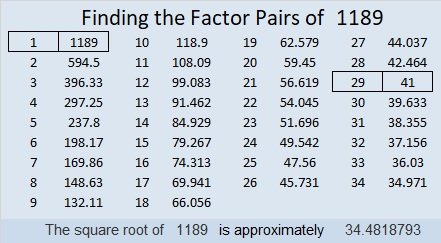# 1189 and Level 2

Some of the clues in this level 2 puzzle were also in the level 1 puzzle earlier this week. Can you remember their common factors and figure out the common factors for the other three sets of clues?Print the puzzles or type the solution in this excel file: 12 factors 1187-1198

Here are some facts about the number 1189:

• 1189 is a composite number.
• Prime factorization: 1189 = 29 × 41
• The exponents in the prime factorization are 1 and 1. Adding one to each and multiplying we get (1 + 1)(1 + 1) = 2 × 2 = 4. Therefore 1189 has exactly 4 factors.
• Factors of 1189: 1, 29, 41, 1189
• Factor pairs: 1189 = 1 × 1189 or 29 × 41
• 1189 has no square factors that allow its square root to be simplified. √1189 ≈ 34.4818833² + 10² = 1189
30² + 17² = 1189

1189 is the hypotenuse of FOUR Pythagorean triples:
261-1160-1189 which is 29 times (9-40-41)
611-1020-1189 calculated from 30² – 17², 2(30)(17), 30² + 17²
660-989-1189 calculated from 2(33)(10), 33² – 10², 33² + 10²
820-861-1189 which is (20-21-29) times 41

1189 is 10010100101 in BASE 2. That’s a nice pattern.
It’s also palindrome 1H1 in base 27 ( H is 17 base 10),
and palindrome 131 in BASE 33